# Python | Create a Voting Classifier using sklearn

In this tutorial, we will focus on how to create a voting classifier using sklearn in Python.

Instead of checking which model predicts better, we can use all the models and combine them using an Ensemble method known as “Voting Classifier” because the combined model always gives better accuracy than the individual.

Pre-requisite:

This post contains:

1. What is Voting classifier?
2. Types of Voting Classifier.
3. Applying this concept using Python’s Scikit-learn library

## VOTING CLASSIFIERTwo types of Voting Classifier:

1. Hard Voting – It takes the majority vote as a final prediction.
2. Soft Voting  – It takes the average of the class probability. (The value above the threshold value as 1, and below the threshold value as 0).

Instantiating Voting Classifier:

In this tutorial, We will implement a voting classifier using Python’s scikit-learn library.

```from sklearn.ensemble import VotingClassifier

clf_voting=VotingClassifier ( estimators=[(string,estimator)], voting)```

Note:

The voting classifier can be applied only to classification problems.

Use an odd number of classifiers(min 3) to avoid a tie.

Here, we will use three different algorithms such as

• SVM
• Logistic Regression
• Decision Tree method

Example: Breast-cancer dataset.

```#Import the necessary libraries

import pandas as pd
import numpy as np

#import the scikit-learn's in-built dataset

```#Have a look at the dataset

cancer_cells.keys()```

Output: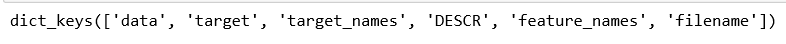`cancer_cells['feature_names']`

Output: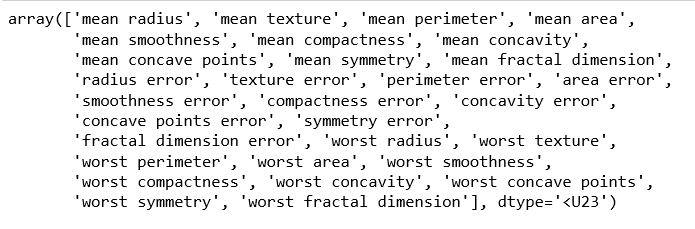`cancer_cells['target_names']`

Output: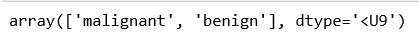`cancer_cells['target']`

Output: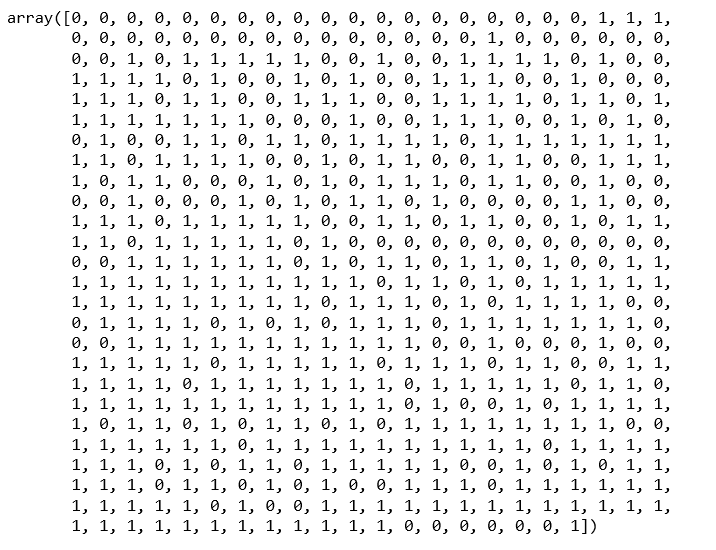```# creating a data frame

cancer_feat = pd.DataFrame(cancer_cells['data'],columns=cancer_cells['feature_names'])

Output: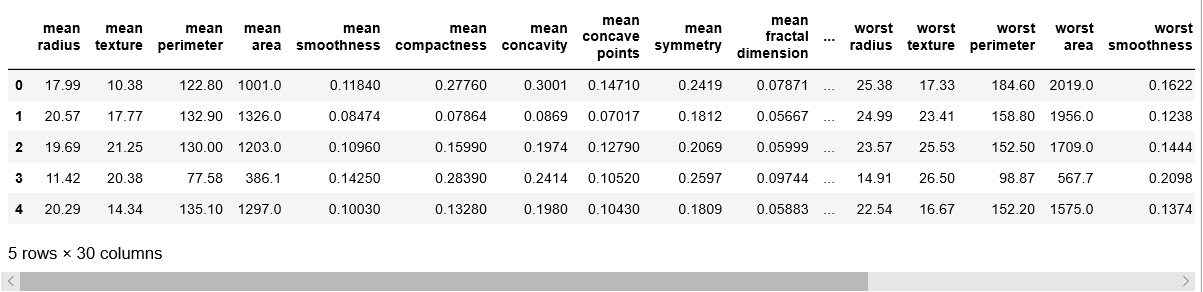```#Splitting into training and testing data

from sklearn.model_selection import train_test_split
X=cancer_feat
y=cancer_cells['target']
X_train, X_test, y_train, y_test = train_test_split(X, y, test_size=0.3, random_state=101)```

Now, Predict using the Support Vector Machine algorithm:

Refer Support Vector Machines for classification of data to know more about SVM.

```#instantiate SVM

from sklearn.svm import SVC
svm=SVC()

#Fit the model to the training dataset

svm.fit(X_train,y_train)

#Predict using the test set

predictions=svm.predict(X_test)

#instantiate Evaluation matrics

from sklearn.metrics import classification_report,confusion_matrix
print(confusion_matrix(y_test,predictions))
print(classification_report(y_test,predictions))
```

Output: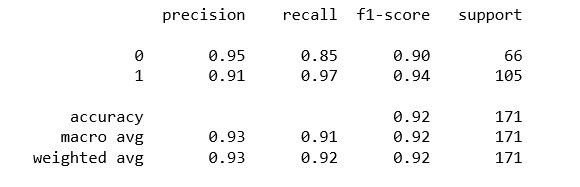Predict using Logistic Regression:

Refer Logistics Regression in Python to know more.

```#Instantiate Logistic Regression

from sklearn.linear_model import LogisticRegression
lr=LogisticRegression()

#Fit the model to the training set and predict using the test set

lr.fit(X_train,y_train)
predictions=lr.predict(X_test)

#Evaluation matrics

print(confusion_matrix(y_test,predictions))
print(classification_report(y_test,predictions))
```

Output: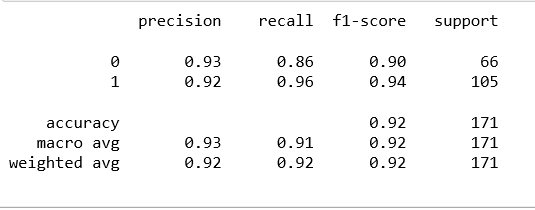Predict using Decision tree method:

```#Instantiate Decision tree model

from sklearn.tree import DecisionTreeClassifier
dt=DecisionTreeClassifier()

#Fit and predict the model

dt.fit(X_train,y_train)
predictions=dt.predict(X_test)

#Evaluation matrics

print(classification_report(y_test,predictions))
```

Output: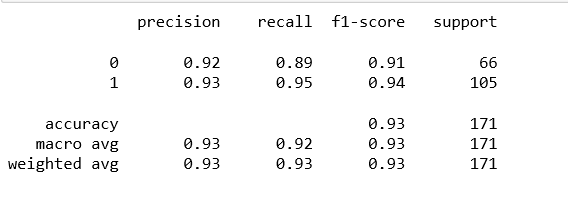Now, Combining all three models using Voting Classifier.

```#import Voting Classifier

from sklearn.ensemble import VotingClassifier

#instantiating three classifiers

logReg= LogisticRegression()
dTree= DecisionTreeClassifier()
svm= SVC()

voting_clf = VotingClassifier(estimators=[('SVC', svm), ('DecisionTree',dTree), ('LogReg', logReg)], voting='hard')

#fit and predict using training and testing dataset respectively

voting_clf.fit(X_train, y_train)
predictions = voting_clf.predict(X_test)

#Evaluation matrics

print(confusion_matrix(y_test,predictions))
print(classification_report(y_test,preds))```

Output: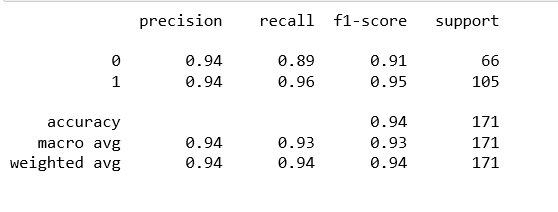Conclusion:

From this, we can observe that by implementing the Voting based Ensemble model, we got the combined accuracy higher than the individual accuracy.

I hope this post helps!